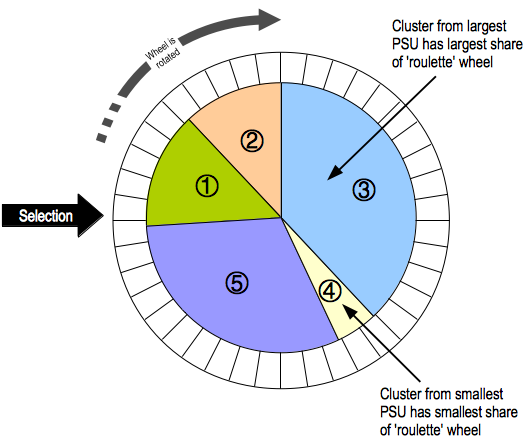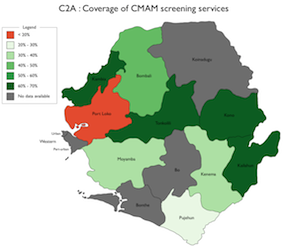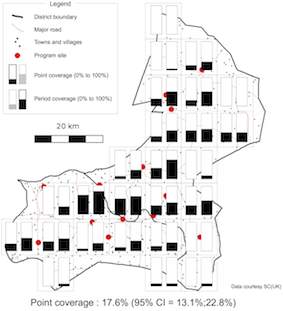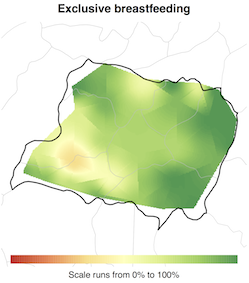Language: English Français

# Coverage survey using Blocked Weighted Bootstrap

This question was posted the Assessment and Surveillance forum area and has 14 replies.

### Mark Myatt

Frequent user

27 Apr 2014, 10:32

The blocked weighted bootstrap (BBW) is an estimation technique for use with data from two-stage cluster sampled surveys in which either prior weighting (e.g. PPS as used in SMART surveys) or posterior weighting (e.g. as used in RAM and S3M surveys). The method was developed by ACF, Brixton Health, CONCERN, GAIN, UNICEF (Sierra Leone), UNICEF (Sudan) and VALID. It has been tested by CDC using IYCF data. The bootstrap technique is summarised in this Wikipedia article. The BWB used in RAM and S3M is a modification to the percentile bootstrap to include blocking and weighing to account for a complex sample design. With RAM and S3M surveys, the sample is complex in the sense that it is an unweighted cluster sample. Data analysis procedures need to account for the sample design. A blocked weighted bootstrap (BWB) can be used : Blocked : The block corresponds to the primary sampling unit (PSU = cluster). PSUs are resampled with replacement. Observations within the resampled PSUs are also sampled with replacement. Weighted : RAM and S3M samples do not use population proportional sampling (PPS) to weight the sample prior to data collection (e.g. as is done with SMART surveys). This means that a posterior weighting procedure is required. BBW uses a "roulette wheel" algorithm (see illustration below) to weight (i.e. by population) the selection probability of PSUs in bootstrap replicates.In the case of prior weighting by PPS all clusters are given the same weight. With posterior weighting (as in RAM or S3M) the weight is the population of each PSU. This procedure is very similar to the fitness proportional selection technique used in evolutionary computing. A total of m PSUs are sampled with replacement for each bootstrap replicate (where m is the number of PSUs in the survey sample). The required statistic is applied to each replicate. The reported estimate consists of the 0.025th (95% LCL), 0.5th (point estimate), and 0.975th (95% UCL) quantiles of the distribution of the statistic across all survey replicates. Early versions of the BBW did not resample observations within PSUs following : ``` Cameron AC, Gelbach JB, Miller DL, Bootstrap-based improvements for inference with clustered errors, Review of Economics and Statistics, 2008:90;414–427 ``` and used a large number (e.g. 3999) survey replicates. Current versions of the BBW resample observations within PSUs and use a smaller number of survey replicates (e.g. n = 400). This is a more computationally efficient approach The BBW has been implemented in the R language for Data Analysis and Graphics. The curent code (as of 21/03/2014) for the BBW is available here. This code usually forms part of a larger survey analysis workflow. I am happy to help you get this to work. BTW : I think an unweighted cluster sample using a spatial sample is the best approach to estimating coverage. I hope this is of some use.

### Mark Myatt

Frequent user

28 Apr 2014, 11:45

I forgot to mention ... For most survey analysis needs (e.g. estimating means and proportions) you can use model-based estimation techniques instead of the BBW (or nay resampling technique). These are provided in standard statistical packages (e.g. SPSS Complex Samples module, EpiInfo CSAMPLE module, STATA "svy" commands, R / S+ "survey" library, SAS via SUDAAN) as well as in specialised complex sample survey analysis systems such as SUDAAN. The main reason to use BBW is that the bootstrap allows a wider range statistics to be calculated than model-based techniques without resort to grand assumptions about the sampling distribution of the required statistic. A good example for this is the confidence interval on the difference between two medians which might be used for many socio-economic variables. The BBW also allows for a wider range of hypothesis tests to be used with complex sample survey data. I like the bootstrap because of its "fixed complexity" (with the bootstrap all statistical questions are equally simple), improved accuracy over most model-based techniques, versatility (see above), and the ability to make inferences using small (i.e. smaller than commonly used with classical statistical procedures) sample sizes. If you only need to (e.g.) calculate coverage proportions and rank barriers to coverage then you could analyse your survey data using one of the packages mentioned above. I hope this helps.

### Mark Myatt

Frequent user

1 May 2014, 08:16

Glad to be of help. Do not hesitate to contact me (via this forum or directly) should you need any help with this.

### Mark Myatt

Frequent user

29 May 2014, 08:49

There are three approaches that I use. The first is to use a set of RAM surveys. One survey per district. This provides mapping at the district level. Here is an example from Sierra Leone:For this map ... data were imported into R, indicators were created from collected variables, and the coverage proportion estimated. The point estimate of the coverage proportion was mapped. The map above was drawn in OpenOffice Draw but R could have been used to produce a similar map automatically. The per-district RAM approach gives similar output in terms of mapping resolution as you might get from per-district SMART surveys or from wide-area surveys such as MICS or DHS. A related method is SLEAC. This uses a small sample size (i.e. n = 40) and maps coarse coverage classes (e.g. < 20%, 20% - 50%, > 50%). Software for analysing and reporting on RAM survey data is available. This is a customisable workflow system. Customising this software requires some knowledge of R. Better resolution can be achieved using the CSAS approach that was used when we were developing CTC (we now call it CMAM). This is about as simple as a spatial survey method can get. Here is an example of CSAS output for CMAM coverage:The data for this map were collated by hand on a tally sheet. Estimates were calculated in a spreadsheet. The map was made using OpenOffice Draw. Software (in Excel and in R) is available that produces maps directly from the data. CSAS is so simple that analysis and mapping can be (and has been) done by hand. A less simple but much improved version of CSAS has been developed by a number of partners (i.e. Brixton Health, VALID, EHNRI, CONCERN, GAIN, and UNICEF). This is known as S3M. Resolution is much better than is practicable with CSAS and data are used more intensively. Here is an example S3M map showing EBF coverage in several districts in Ethiopia:This map was produced in R using S3M survey data and ARCGIS boundary files. I do not want to go into the exact procedures for doing this in R. I do not feel that this is the proper place for this as R is well supported elsewhere with sites that specifically support using R for geo-statistical analysis. The procedure is quite straightforward if you are familiar with R and the way R handles spatial objects (there is a book in the "useR!" series). Free and open-source customisable survey software for S3M is available. This requires a familiarity with the R language (there are many books including one written by me). The common mode of working is for survey data to be analysed and mapped during a training course which teaches using R for epidemiological work and then customises the S3M survey system to produce the indicators and maps required. You may want to consider this approach. From your previous posts I think that you would be using a per-district RAM approach. I think the easiest approach would be to use the R workflow to manage and analyse data and then map by hand using a low-end / entry-level GIS system (e.g. ArcView ... now called ArcGIS BASIC) or a vector graphics program such as OpenOffice Draw. I hope this helps.

### Ernest Guevarra

Katilingban

Frequent user

2 Jun 2014, 07:42

### Mark Myatt

Frequent user

2 Jun 2014, 14:49

Thanks for picking this up.

### Mark Myatt

Frequent user

29 Sep 2015, 08:51

First let us unpack the term "block weighted bootstrap". The "block" and weighted parts refer to the way a bootstrap replicate (a "pseudo-survey" created by resampling the real data) is created. For survey work this replicates the sampling method. With a SMART type survey we used a blocked method and the replicate sample is taken as a sample with replacement of the clusters and then a sample with replacement from with the cluster. There is on weighting because a SMART survey uses PPS to prior weight the sample. For a RAM or S3M sample (both use a spatial sampling) the replicates is made by sampling clusters with replacement and proportional to population sizes. You question is more about the "bootstrap" part of the term. the method is very simple. Using your example: (1) Take r = 500 replicates from the baseline survey. Calculate prevalence in each replicates. This will give you r = 500 baseline prevalences. Let us call this BP. (2) Take r = 500 replicates from the endline survey. Calculate prevalence in each replicates. This will give you r = 500 endline prevalences. Let us call this EP. (3) You are interested in the difference between the baseline and endline prevalence. We can estimate this by subtracting the replicate prevalences from each other: ``` Replicate BP EP Difference (BP - EP) ------------ ----- ----- -------------------- 1 0.121 0.105 0.016 2 0.133 0.114 0.019 3 0.125 0.129 -0.004 4 0.091 0.113 -0.022 . . . . . . . . . . . . 500 0.120 0.112 0.008 ------------ ----- ----- -------------------- ``` (4) There are two ways to proceed from here. If you are interested in estimating the magnitude of the difference then find the MEDIAN difference (this is the point estimate of the difference) and the 2.5th percentile and the 97.5th percentile (these are the lower and upper 95% confidence limits of the difference). If the confidence interval contains zero then you might conclude that there is no significant difference. If you want a p-value then count the number of differences that are less than or equal to zero and divide that by the number of replicates. If (e.g.) there were 11 differences <= 0 and 500 replicates then p = 11 / 500 = 0.0220. I present here an example coded in R of a standard bootstrap example related to weight gain in two groups of pigs on different dietary supplements: ``` # # The weight gains on the two diets # diet1 <- c(31, 34, 29, 26, 32, 35, 38, 34, 31, 29, 32, 31) diet2 <- c(26, 24, 28, 29, 30, 29, 31, 29, 32, 26, 28, 32) # # Accumulator for the differences # differences <- NULL # # Take 500 replicates # for(i in 1:500) { # # Replicates are mean weight gains on each diet # r1 <- mean(sample(diet1, replace = TRUE)) r2 <- mean(sample(diet2, replace = TRUE)) # # Differences # differences <- c(differences, r1 - r2) } # # Estimates # quantile(differences, probs = c(0.5, 0.025, 0.975)) # # A p-value # z <- ifelse(differences <= 0, 1, 0) sum(z) / 500 ``` When I run this I got difference = 3.17 (95% CI = 1.08; 5.58) with p = 0.0020. Similar results can be obtained using a simple t-test. You may ask "Why use the bootstrap?" ... several answers: (1) It is very efficient WRT sample size. (2) It is (in the form given above) non-parametric using empirical rather than theoretical distributions. There are no assumptions of (e.g.) normality to violate. (3) We can use any statistic we want. There is (e.g.) no classical test for differences in medians. For the bootstrap above we can do this by replacing "mean" with "median". We could easily have looked at total weight gain by replacing "mean" with "sum". Classical test are limited to a few statistics. (4) What you see above is as complicated as it gets. Classical test can get complicated quite quickly. Anyway ... I hope this helps.